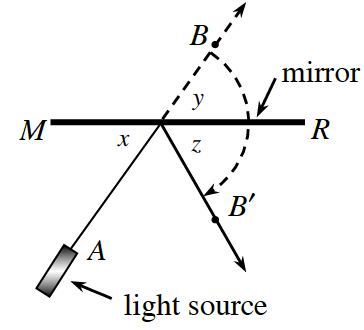### Home > INT2 > Chapter 2 > Lesson 2.3.2 > Problem2-95

2-95.

A laser light is pointed at a mirror as shown at right. If $∠x$ measures $48^\circ$, what are the measures of $∠y$ and $∠z$? Justify your reasons.

Angle $x$ and angle $y$ are separated by a vertical angle. Does that say anything about their value? Also, angle $z$ is a reflection angle.

$y=48^\circ$ because vertical angles are equal.
$z=48^\circ$ because the angle of incidence equals the angle of reflection.